# HCF - Highest Common Factor

HCF or Highest Common Factor is the greatest number which divides each of the two or more numbers. HCF is also called the Greatest Common Measure (GCM) and Greatest Common Divisor(GCD). HCM and LCM are two different methods, where LCM or Least Common Multiple is used to find the smallest common multiple of any two or more numbers.

## What is HCF?

The full form of HCF is Highest Common Factor. HCF of two numbers is the highest factor that can divide the two numbers, evenly. HCF can be evaluated for two or more than two numbers.  It is the greatest divisor for any two or more numbers, that can equally or completely divide the given numbers.

For Example: The Highest common factor of 60 and 75 is 15 because 15 is the largest number which can divide both 60 and 75 exactly.

## How to Find HCF?

We can find the HCF of any given numbers by using two methods:

• by prime factorization method
• by division method

Let us discuss these two methods one by one in this article.

### HCF By Prime Factorization Method

Follow the below-given steps to find the HCF of numbers using the prime factorisation method.

• Step 1: Write each number as a product of its prime factors. This method is called here prime factorization.
• Step 2: Now list the common factors of both the numbers
• Step 3: The product of all common prime factors is the HCF ( use the lower power of each common factor)

Let us understand with the help of examples.

Example 1: Evaluate the HCF of 60 and 75.

Solution:

 Write each number as a product of its prime factors. 22 x 3 x 5 = 60 3 x 52 = 75 The product of all common prime factors is the HCF. The common prime factors in this example are 3 & 5. The lowest power of 3 is 3 and 5 is 5. So, HCF = 3 x 5 = 15

Example 2: Find the HCF of 36, 24 and 12.

Solution:

 Write each number as a product of its prime factors. 22 x 32 = 36 23 x 3 = 24 22 x 3 = 12 The product of all common prime factors is the HCF ( use the lowest power of each common factor) The common prime factors in this example are 2 & 3. The lowest power of 2 is 22 and 3 is 3. So, HCF = 22 x 3 = 12

Example 3: Find the HCF of 36, 27 and 80.

Solution:

 Write each number as a product of its prime factors. 22 x 32 = 36 33 = 27 24 x 5 = 80 The product of all common prime factors is the HCF The common prime factors in this example are none. So, HCF is 1.

### HCF By Division Method

You have understood by now the method of finding the highest common factor using prime factorization. Now let us learn here to find HCF using the division method. Basically, the division method is nothing but dividing the given numbers, simultaneously, to get the common factors between them. Follow the steps mentioned below to solve the problems of HCF.

• Step 1: Write the given numbers horizontally, in a sequence, by separating them with commas.
• Step 2: Find the smallest prime number which can divide the given number. It should exactly divide the given numbers. (Write on the left side).
• Step 3: Now write the quotients.
• Step 4: Repeat the process, until you reach the stage, where there is no coprime number left.
• Step 5: We will get the common prime factors as the factors on the left-hand side divides all the numbers exactly. The product of these common prime factors is the HCF of the given numbers.

Let us understand the above-mentioned steps to find the HCF by division method with the help of examples.

Problem 1: Evaluate the HCF of 30 and 75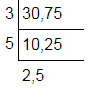As we can note that the mentioned prime factors, on the left side, divide all the numbers exactly. So, they all are common prime factors. We have no common prime factor for the numbers remaining at the bottom.

So, HCF = 3 × 5 = 15.

Example 2: Find out HCF of 36, and 24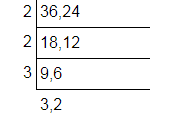HCF = 2 × 2 × 3 = 12.

Example 3: Find out HCF of 36, 12, 24 and 48.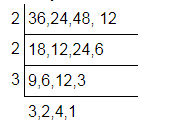HCF = 2 × 2 × 3 = 12.

## HCF by Shortcut method

Steps to find the HCF of any given set of numbers.

• Step 1: Divide the larger number by the smaller number first, such as;

Larger Number/Smaller Number

• Step 2: Divide the divisor of step 1 by the remainder left.

Divisor of step 1/Remainder

• Step 3: Again divide the divisor of step 2 by the remainder.

Divisor of step 2/Remainder

• Step 4: Repeat the process until the remainder is zero.
• Step 5: The divisor of the last step is the HCF.

## How to find the HCF of Three numbers

Find the steps to calculate the HCF of the 3 numbers given to us.

 1) Calculate the HCF of 2 numbers. 2) Then Find the HCF of the 3rd number and the HCF found in step 1. 3) The HCF you got in step 2 will be the HCF of the 3 numbers.

The above steps can also be used to find the HCF of more than 3 numbers.

## HCF of Four Numbers

The simple steps to find the HCF of 4 numbers are:

• First, divide the four numbers into pairs of two
• Find the HCF of pair of two numbers
• Then, find the HCF of HCFs calculated in the previous step
• The final HCF will be the required HCF of 4 numbers

## HCF of Prime Numbers

HCF of any two or prime numbers is always equal to 1.

Why?

Because prime numbers are those numbers that have only two factors, either 1 or the number itself. So, if there is no other factor, then the HCF of such numbers will be equal to 1. Find the examples below:

• HCF (2, 3) = 1
• HCF (3, 7) = 1
• HCF (5, 13) = 1
• HCF (19, 23) = 1

## Properties of HCF

• HCF of two or more numbers is the divides the given numbers, exactly
• HCF of prime numbers is always equal to 1
• HCF is always less than or equal to the given numbers

## HCF Solved Examples

Here is a few more example to find the highest common factors.

Example 1: Find out HCF of 30 and 45.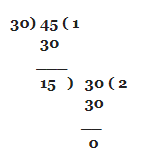So, the HCF of 30 and 45 is 15.

Example 2: Find out HCF of 12 and 36.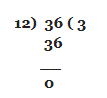So, HCF of 12 and 36 = 12

Example 3: Find out HCF of 9, 27, and 30

Take any two numbers and find out their HCF first. Say, let’s find out HCF of 9 and 27 initially.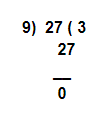So, HCF of 9 and 27 = 9

HCF of 9 ,27, 30

= HCF of [(HCF of 9, 27) and 30

= HCF of [9 and 30]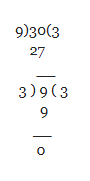Hence, HCF of 9 ,27, 30 = 3

Example 4: Find out HCF of 5 and 7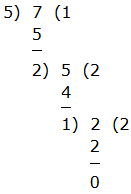Hence, HCF of 5 and 7 = 1

Example 5: 2 given numbers are in the ratio 5:11. One of the HCF is number 7, what are the numbers?

Assume the numbers to be 5m & 11m.

Here “m” is the highest common factor (HCF).

The numbers are 5 × 7 = 35 and 11 × 7 = 77.

## Word Problems on HCF

Q.1: Determine the plank length that can be utilised to measure the given values of length 4 m 50 cm, 9 m 90 cm and 16 m 20 cm in the limited time period.

On conversion of length to centimeters,

4 m 50 cm = 450 cm

9 m 90 cm = 990 cm

16 m 20 cm = 1620 cm

To determine the length of the sizeable plank that can be utilised to measure the given values of length in a limited time period, the highest common factor needs to be taken.

450 = 2 × 3 × 3 × 5 × 5 = 2 × 32 × 52

990 = 2 × 3 × 3 × 5 × 11 = 2 × 32 × 5 × 11

1620 = 2 × 2 × 3 × 3 × 3 × 3 × 5 = 22 × 34 × 5

HCF (450, 990, 1620) = 2 × 3 × 3 × 5 = 90

Q.2: Determine the largest number on dividing 70 and 50 leaves behind remainders one and four.

The essential digit leaves behind remainders one and four on dividing 70 and 50.

The numbers 69 and 46 are obtained.

To determine the HCF 69 (3 × 23) and 46 (2 × 23).

The highest common factor (HCF) of 69 and 46 is 23.

Q.3: Determine the greatest number that divides 64, 136 and 238 and leaves behind the same remainder in every case.

To determine the required number, the highest common factor of (136 – 64), (238 – 136) and (238 – 64) that is the HCF (72, 102, 174) needs to be found.

72 = 23 × 32

102 = 2 × 3 × 17

174 = 2 × 3 × 29

HCF (72, 102, 174) = 2 × 3 = 6

6 is the required number.

Q.4: Find the highest count of students among whom 182 chocolates and 247 candies can be given such that every student obtains the same count of each. Also, what is the count of the chocolates and candies every student will obtain?

The value of HCF of the count of chocolates and candies that needs to be found gives the count of the students.

HCF (182, 247) = 13

13 students.

Count of chocolates for every student = 182/13 = 14

Count of candies for every student = 247/13 = 19

### Practice Problems

1. What is the HCF of three numbers, 43, 91 and 183?
2. The product of the two numbers is 4107. If the HCF of these numbers is 37, then find the greater number.
3. Calculate the HCF of 36 and 84 by the prime factorization method.

Learn more on these mathematical topics by subscribing to BYJU’S – The Learning App.

## Frequently Asked Questions on HCF

Q1

### What is meant by HCF in Maths?

In Maths, HCF means the Highest Common Factor. When finding the factors of two or more numbers, some numbers are found to be common. The greatest factor found from the common factors is called the HCF. Sometimes, it is also called the greatest common factor.

Q2

### Mention the different methods to calculate the HCF of numbers?

The two most common methods to find the HCF of the given numbers are:
Prime Factorization Method
Division Method

Q3

### How to calculate the HCF of 3 numbers?

The procedure to find the HCF of 3 numbers is:
Step 1: Find the HCF of any two given numbers
Step 2: Now, find the HCF of the remaining number and the result obtained from step 1.
Step 3: The HCF of the 3 numbers is the result obtained from step 2.

Q4

### How to find the HCF of the numbers?

Step 1: Write down each number as the product of its prime factors.
Step 2: Now, list down the common factors from the given numbers.
Step 3: The largest number, which is found in the common factors is the HCF of the given numbers.

Q5

### What is the HCF of 20 and 34?

The prime factors of 20 are 1x 2x 2 x5
The prime factors of 34 are 1x 2 x 17
Here, 2 is the common factor obtained from both numbers. Hence, the HCF of 20 and 34 is 2.